# Probabilistic Programming and Bayesian Methods for Hackers Chapter 6¶

Original content (this Jupyter notebook) created by Cam Davidson-Pilon (@Cmrn_DP)

Ported to Tensorflow Probability by Matthew McAteer (@MatthewMcAteer0), with help from Bryan Seybold, Mike Shwe (@mikeshwe), Josh Dillon, and the rest of the TFP team at Google ([email protected]).

Welcome to Bayesian Methods for Hackers. The full Github repository is available at github/Probabilistic-Programming-and-Bayesian-Methods-for-Hackers. The other chapters can be found on the project's homepage. We hope you enjoy the book, and we encourage any contributions!

### Table of Contents¶

• Dependencies & Prerequisites
• Getting our priorities straight
• Subjective vs Objective priors
• Subjective Priors
• Decisions, decisions...
• Empirical Bayes
• Useful priors to know about
• The Gamma distribution
• The Wishart distribution
• The Beta distribution
• Example: Bayesian Multi-Armed Bandits
• Applications
• A Proposed Solution
• A Measure of Good
• Extending the algorithm
• Eliciting expert prior
• Trial roulette method
• Example: Stock Returns
• Protips for the Wishart distribution
• Conjugate Priors
• Jeffreys Priors
• Effect of ther prior as N increases
• Bayesian perspective of Penalized Linear Regressions
• References

This chapter of Bayesian Methods for Hackers focuses on the most debated and discussed part of Bayesian methodologies: how to choose an appropriate prior distribution. We also present how the prior's influence changes as our dataset increases, and an interesting relationship between priors and penalties on linear regression.

### Dependencies & Prerequisites¶

Tensorflow Probability is part of the colab default runtime, so you don't need to install Tensorflow or Tensorflow Probability if you're running this in the colab.
If you're running this notebook in Jupyter on your own machine (and you have already installed Tensorflow), you can use the following
• For the most recent nightly installation: pip3 install -q tfp-nightly
• For the most recent stable TFP release: pip3 install -q --upgrade tensorflow-probability
• For the most recent stable GPU-connected version of TFP: pip3 install -q --upgrade tensorflow-probability-gpu
• For the most recent nightly GPU-connected version of TFP: pip3 install -q tfp-nightly-gpu
Again, if you are running this in a Colab, Tensorflow and TFP are already installed
In :
#@title Imports and Global Variables  { display-mode: "form" }
"""
The book uses a custom matplotlibrc file, which provides the unique styles for
matplotlib plots. If executing this book, and you wish to use the book's
styling, provided are two options:
1. Overwrite your own matplotlibrc file with the rc-file provided in the
book's styles/ dir. See http://matplotlib.org/users/customizing.html
2. Also in the styles is  bmh_matplotlibrc.json file. This can be used to
update the styles in only this notebook. Try running the following code:

import json
s = json.load(open("../styles/bmh_matplotlibrc.json"))
matplotlib.rcParams.update(s)
"""
!pip3 install -q pandas_datareader
!pip3 install -q wget
from __future__ import absolute_import, division, print_function

#@markdown This sets the warning status (default is ignore, since this notebook runs correctly)
warning_status = "ignore" #@param ["ignore", "always", "module", "once", "default", "error"]
import warnings
warnings.filterwarnings(warning_status)
with warnings.catch_warnings():
warnings.filterwarnings(warning_status, category=DeprecationWarning)
warnings.filterwarnings(warning_status, category=UserWarning)

import numpy as np
import os
#@markdown This sets the styles of the plotting (default is styled like plots from [FiveThirtyeight.com](https://fivethirtyeight.com/))
matplotlib_style = 'fivethirtyeight' #@param ['fivethirtyeight', 'bmh', 'ggplot', 'seaborn', 'default', 'Solarize_Light2', 'classic', 'dark_background', 'seaborn-colorblind', 'seaborn-notebook']
import matplotlib.pyplot as plt; plt.style.use(matplotlib_style)
import matplotlib.axes as axes;
from matplotlib.patches import Ellipse
import scipy.stats as stats
rand = np.random.rand
beta = stats.beta
from mpl_toolkits.mplot3d import Axes3D
import pandas_datareader.data as web
%matplotlib inline

import seaborn as sns; sns.set_context('notebook')
from IPython.core.pylabtools import figsize
#@markdown This sets the resolution of the plot outputs (retina is the highest resolution)
notebook_screen_res = 'retina' #@param ['retina', 'png', 'jpeg', 'svg', 'pdf']
%config InlineBackend.figure_format = notebook_screen_res

import tensorflow as tf
tfe = tf.contrib.eager

# Eager Execution
#@markdown Check the box below if you want to use [Eager Execution](https://www.tensorflow.org/guide/eager)
#@markdown Eager execution provides An intuitive interface, Easier debugging, and a control flow comparable to Numpy. You can read more about it on the [Google AI Blog](https://ai.googleblog.com/2017/10/eager-execution-imperative-define-by.html)
use_tf_eager = False #@param {type:"boolean"}

# Use try/except so we can easily re-execute the whole notebook.
if use_tf_eager:
try:
tf.enable_eager_execution()
except:
pass

import tensorflow_probability as tfp
tfd = tfp.distributions
tfb = tfp.bijectors

def evaluate(tensors):
"""Evaluates Tensor or EagerTensor to Numpy ndarrays.
Args:
tensors: Object of Tensor or EagerTensors; can be list, tuple,
namedtuple or combinations thereof.

Returns:
ndarrays: Object with same structure as tensors except with Tensor or
EagerTensors replaced by Numpy ndarrays.
"""
if tf.executing_eagerly():
return tf.contrib.framework.nest.pack_sequence_as(
tensors,
[t.numpy() if tf.contrib.framework.is_tensor(t) else t
for t in tf.contrib.framework.nest.flatten(tensors)])
return sess.run(tensors)

class _TFColor(object):
"""Enum of colors used in TF docs."""
red = '#F15854'
blue = '#5DA5DA'
orange = '#FAA43A'
green = '#60BD68'
pink = '#F17CB0'
brown = '#B2912F'
purple = '#B276B2'
yellow = '#DECF3F'
gray = '#4D4D4D'
def __getitem__(self, i):
return [
self.red,
self.orange,
self.green,
self.blue,
self.pink,
self.brown,
self.purple,
self.yellow,
self.gray,
][i % 9]
TFColor = _TFColor()

def session_options(enable_gpu_ram_resizing=True, enable_xla=True):
"""
Allowing the notebook to make use of GPUs if they're available.

XLA (Accelerated Linear Algebra) is a domain-specific compiler for linear
algebra that optimizes TensorFlow computations.
"""
config = tf.ConfigProto()
config.log_device_placement = True
if enable_gpu_ram_resizing:
# allow_growth=True makes it possible to connect multiple colabs to your
# GPU. Otherwise the colab malloc's all GPU ram.
config.gpu_options.allow_growth = True
if enable_xla:
# Enable on XLA. https://www.tensorflow.org/performance/xla/.
config.graph_options.optimizer_options.global_jit_level = (
tf.OptimizerOptions.ON_1)
return config

def reset_sess(config=None):
"""
Convenience function to create the TF graph & session or reset them.
"""
if config is None:
config = session_options()
global sess
tf.reset_default_graph()
try:
sess.close()
except:
pass
sess = tf.InteractiveSession(config=config)

reset_sess()

  Building wheel for wget (setup.py) ... done


The default version of TensorFlow in Colab will soon switch to TensorFlow 2.x.
We recommend you upgrade now or ensure your notebook will continue to use TensorFlow 1.x via the %tensorflow_version 1.x magic: more info.

WARNING:tensorflow:
The TensorFlow contrib module will not be included in TensorFlow 2.0.
For more information, please see:
* https://github.com/tensorflow/community/blob/master/rfcs/20180907-contrib-sunset.md
* https://github.com/tensorflow/addons
* https://github.com/tensorflow/io (for I/O related ops)
If you depend on functionality not listed there, please file an issue.

Device mapping:
/job:localhost/replica:0/task:0/device:XLA_CPU:0 -> device: XLA_CPU device



## Getting our priorities straight¶

Up until now, we have mostly ignored our choice of priors. This is unfortunate as we can be very expressive with our priors, but we also must be careful about choosing them. This is especially true if we want to be objective, that is, not to express any personal beliefs in the priors.

### Subjective vs Objective priors¶

Bayesian priors can be classified into two classes: objective priors, which aim to allow the data to influence the posterior the most, and subjective priors, which allow the practitioner to express his or her views into the prior.

What is an example of an objective prior? We have seen some already, including the flat prior, which is a uniform distribution over the entire possible range of the unknown. Using a flat prior implies that we give each possible value an equal weighting. Choosing this type of prior is invoking what is called "The Principle of Indifference", literally we have no prior reason to favor one value over another. Calling a flat prior over a restricted space an objective prior is not correct, though it seems similar. If we know $p$ in a Binomial model is greater than 0.5, then $\text{Uniform}(0.5,1)$ is not an objective prior (since we have used prior knowledge) even though it is "flat" over [0.5, 1]. The flat prior must be flat along the entire range of possibilities.

Aside from the flat prior, other examples of objective priors are less obvious, but they contain important characteristics that reflect objectivity. For now, it should be said that rarely is a objective prior truly objective. We will see this later.

#### Subjective Priors¶

On the other hand, if we added more probability mass to certain areas of the prior, and less elsewhere, we are biasing our inference towards the unknowns existing in the former area. This is known as a subjective, or informative prior. In the figure below, the subjective prior reflects a belief that the unknown likely lives around 0.5, and not around the extremes. The objective prior is insensitive to this.

In :
plt.figure(figsize(12.5, 7))

colors = [TFColor, TFColor, TFColor, TFColor]

x = tf.linspace(start=0., stop=1., num=50)
obj_prior_1 = tfd.Beta(1., 1.).prob(x)
subj_prior_1 = tfd.Beta(10., 10.).prob(x)
subj_prior_2 = 2 * tf.ones(25)

[
x_, obj_prior_1_, subj_prior_1_, subj_prior_2_,
] = evaluate([
x, obj_prior_1, subj_prior_1, subj_prior_2,
])

p = plt.plot(x_, obj_prior_1_,
label='An objective prior \n(uninformative, \n"Principle of Indifference")')
plt.fill_between(x_, 0, obj_prior_1_, color = p.get_color(), alpha = 0.3)

p = plt.plot(x_, subj_prior_1_ ,
label = "A subjective prior \n(informative)")
plt.fill_between(x_, 0, subj_prior_1_, color = p.get_color(), alpha = 0.3)

p = plt.plot(x_[25:], subj_prior_2_,
label = "another subjective prior")
plt.fill_between(x_[25:], 0, 2, color = p.get_color(), alpha = 0.3)

plt.ylim(0,4)

plt.ylim(0, 4)
leg = plt.legend(loc = "upper left")
leg.get_frame().set_alpha(0.4)
plt.title("Comparing objective vs. subjective priors for an unknown probability");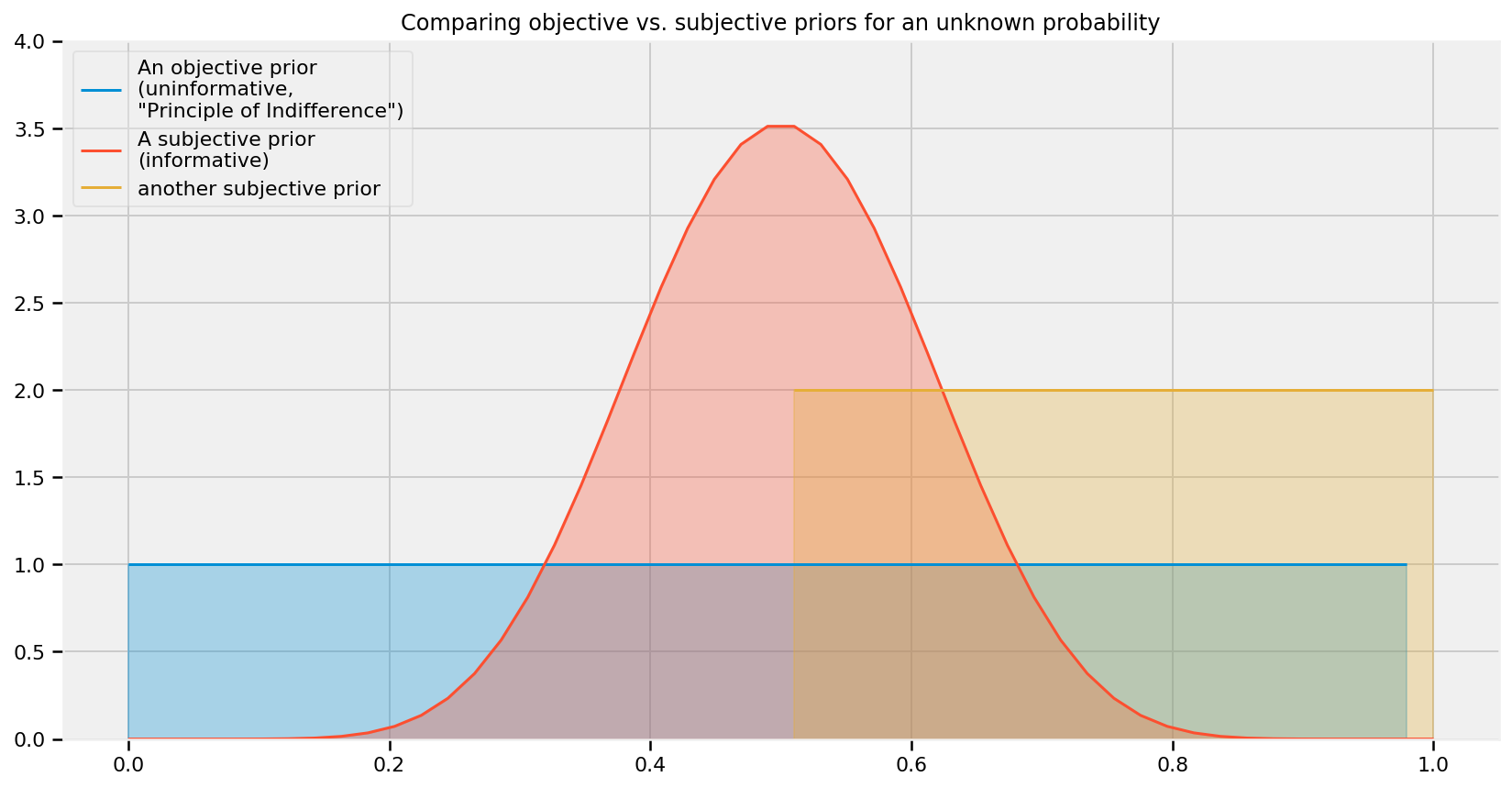The choice of a subjective prior does not always imply that we are using the practitioner's subjective opinion: more often the subjective prior was once a posterior to a previous problem, and now the practitioner is updating this posterior with new data. A subjective prior can also be used to inject domain knowledge of the problem into the model. We will see examples of these two situations later.

### Decision, decisions...¶

The choice, either objective or subjective mostly depends on the problem being solved, but there are a few cases where one is preferred over the other. In instances of scientific research, the choice of an objective prior is obvious. This eliminates any biases in the results, and two researchers who might have differing prior opinions would feel an objective prior is fair. Consider a more extreme situation:

A tobacco company publishes a report with a Bayesian methodology that retreated 60 years of medical research on tobacco use. Would you believe the results? Unlikely. The researchers probably chose a subjective prior that too strongly biased results in their favor.

Unfortunately, choosing an objective prior is not as simple as selecting a flat prior, and even today the problem is still not completely solved. The problem with naively choosing the uniform prior is that pathological issues can arise. Some of these issues are pedantic, but we delay more serious issues to the Appendix of this Chapter.

We must remember that choosing a prior, whether subjective or objective, is still part of the modeling process. To quote Gelman :

... after the model has been fit, one should look at the posterior distribution and see if it makes sense. If the posterior distribution does not make sense, this implies that additional prior knowledge is available that has not been included in the model, and that contradicts the assumptions of the prior distribution that has been used. It is then appropriate to go back and alter the prior distribution to be more consistent with this external knowledge.

If the posterior does not make sense, then clearly one had an idea what the posterior should look like (not what one hopes it looks like), implying that the current prior does not contain all the prior information and should be updated. At this point, we can discard the current prior and choose a more reflective one.

Gelman  suggests that using a uniform distribution with large bounds is often a good choice for objective priors. Although, one should be wary about using Uniform objective priors with large bounds, as they can assign too large of a prior probability to non-intuitive points. Ask yourself: do you really think the unknown could be incredibly large? Often quantities are naturally biased towards 0. A Normal random variable with large variance (small precision) might be a better choice, or an Exponential with a fat tail in the strictly positive (or negative) case.

If using a particularly subjective prior, it is your responsibility to be able to explain the choice of that prior, else you are no better than the tobacco company's guilty parties.

### Empirical Bayes¶

While not a true Bayesian method, empirical Bayes is a trick that combines frequentist and Bayesian inference. As mentioned previously, for (almost) every inference problem there is a Bayesian method and a frequentist method. The significant difference between the two is that Bayesian methods have a prior distribution, with hyperparameters $\alpha$, while empirical methods do not have any notion of a prior. Empirical Bayes combines the two methods by using frequentist methods to select $\alpha$, and then proceeds with Bayesian methods on the original problem.

A very simple example follows: suppose we wish to estimate the parameter $\mu$ of a Normal distribution, with $\sigma = 5$. Since $\mu$ could range over the whole real line, we can use a Normal distribution as a prior for $\mu$. How to select the prior's hyperparameters, denoted ($\mu_p, \sigma_p^2$)? The $\sigma_p^2$ parameter can be chosen to reflect the uncertainty we have. For $\mu_p$, we have two options:

Option 1: Empirical Bayes suggests using the empirical sample mean, which will center the prior around the observed empirical mean:

$$\mu_p = \frac{1}{N} \sum_{i=0}^N X_i$$

Option 2: Traditional Bayesian inference suggests using prior knowledge, or a more objective prior (zero mean and fat standard deviation).

Empirical Bayes can be argued as being semi-objective, since while the choice of prior model is ours (hence subjective), the parameters are solely determined by the data.

Personally, I feel that Empirical Bayes is double-counting the data. That is, we are using the data twice: once in the prior, which will influence our results towards the observed data, and again in the inferential engine of MCMC. This double-counting will understate our true uncertainty. To minimize this double-counting, I would only suggest using Empirical Bayes when you have lots of observations, else the prior will have too strong of an influence. I would also recommend, if possible, to maintain high uncertainty (either by setting a large $\sigma_p^2$ or equivalent.)

Empirical Bayes also violates a theoretical axiom in Bayesian inference. The textbook Bayesian algorithm of:

prior $\Rightarrow$ observed data $\Rightarrow$ posterior

is violated by Empirical Bayes, which instead uses

observed data $\Rightarrow$ prior $\Rightarrow$ observed data $\Rightarrow$ posterior

Ideally, all priors should be specified before we observe the data, so that the data does not influence our prior opinions (see the volumes of research by Daniel Kahneman et. al about anchoring).

## Useful priors to know about¶

### The Gamma distribution¶

A Gamma random variable, denoted $X \sim \text{Gamma}(\alpha, \beta)$, is a random variable over the positive real numbers. It is in fact a generalization of the Exponential random variable, that is:

$$\text{Exp}(\beta) \sim \text{Gamma}(1, \beta)$$

This additional parameter allows the probability density function to have more flexibility, hence allowing the practitioner to express his or her subjective priors more accurately. The density function for a $\text{Gamma}(\alpha, \beta)$ random variable is:

$$f(x \mid \alpha, \beta) = \frac{\beta^{\alpha}x^{\alpha-1}e^{-\beta x}}{\Gamma(\alpha)}$$

where $\Gamma(\alpha)$ is the Gamma function, and for differing values of $(\alpha, \beta)$ looks like:

In :
parameters = [(1, 0.5), (9, 2), (3, 0.5), (7, 0.5)]
x = tf.cast(tf.linspace(start=0.001 ,stop=20., num=150), dtype=tf.float32)

plt.figure(figsize(12.5, 7))
for alpha, beta in parameters:
[
y_,
x_
] = evaluate([
tfd.Gamma(float(alpha), float(beta)).prob(x),
x,
])
lines = plt.plot(x_, y_, label = "(%.1f,%.1f)"%(alpha, beta), lw = 3)
plt.fill_between(x_, 0, y_, alpha = 0.2, color = lines.get_color())
plt.autoscale(tight=True)

plt.legend(title=r"$\alpha, \beta$ - parameters");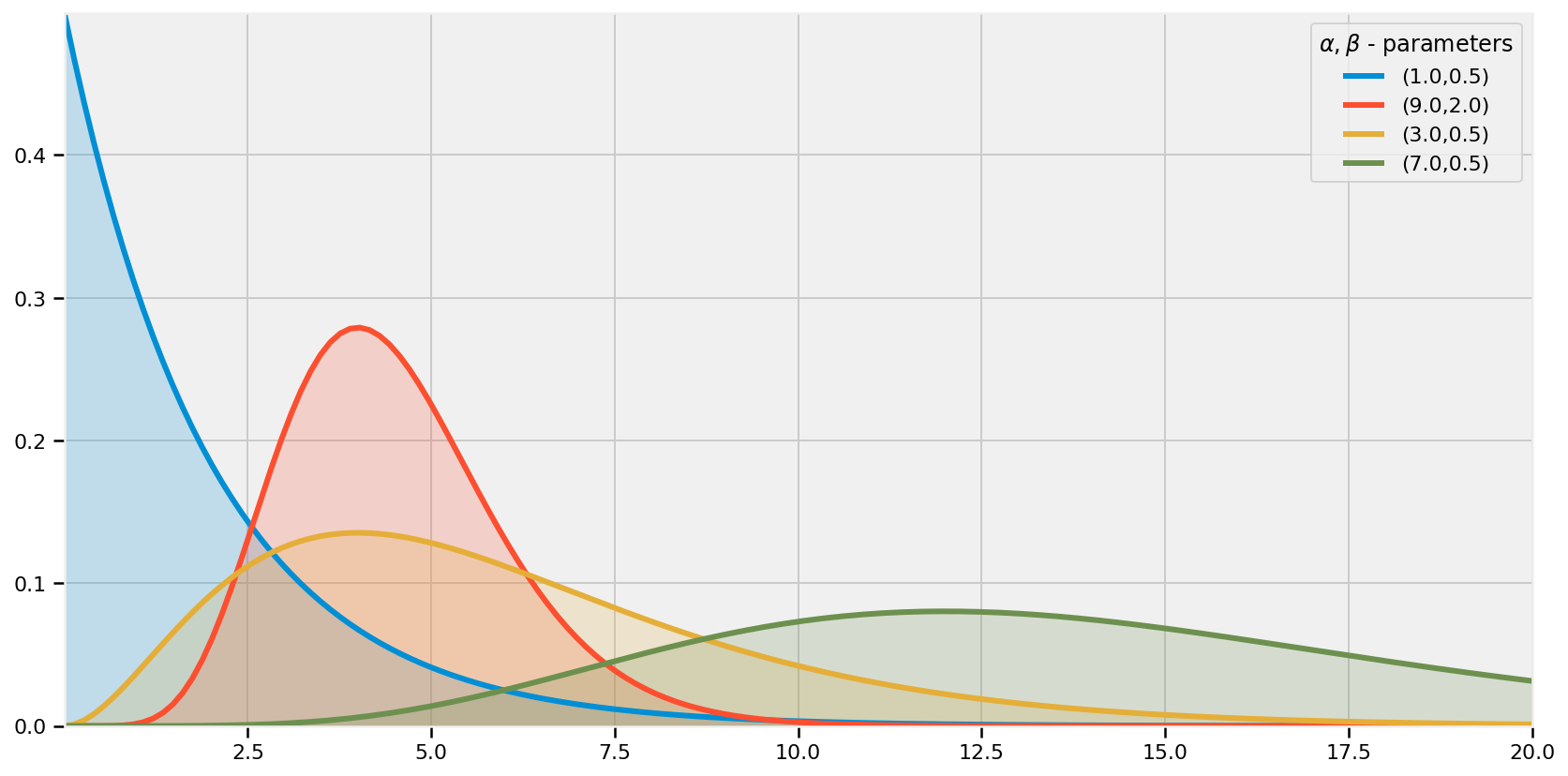### The Wishart distribution¶

Until now, we have only seen random variables that are scalars. Of course, we can also have random matrices! Specifically, the Wishart distribution is a distribution over all positive semi-definite matrices. Why is this useful to have in our arsenal? (Proper) covariance matrices are positive-definite, hence the Wishart is an appropriate prior for covariance matrices. We can't really visualize a distribution of matrices, so I'll plot some realizations from the $5 \times 5$ (above) and $20 \times 20$ (below) Wishart distribution:

In :
reset_sess()

n = 4
print("output of the eye function \n(a commonly used function with Wishart Distributions): \n", np.eye(n))

plt.figure(figsize(12.5, 7))
for i in range(10):
ax = plt.subplot(2, 5, i+1)
if i >= 5:
n = 15
[
wishart_matrices_
] = evaluate([
tfd.Wishart(df=(n+1), scale=tf.eye(n)).sample()
])
plt.imshow( wishart_matrices_,
interpolation="none",
cmap = "hot")
ax.axis("off")

plt.suptitle("Random matrices from a Wishart Distribution");

Device mapping:
/job:localhost/replica:0/task:0/device:XLA_CPU:0 -> device: XLA_CPU device

output of the eye function
(a commonly used function with Wishart Distributions):
[[1. 0. 0. 0.]
[0. 1. 0. 0.]
[0. 0. 1. 0.]
[0. 0. 0. 1.]]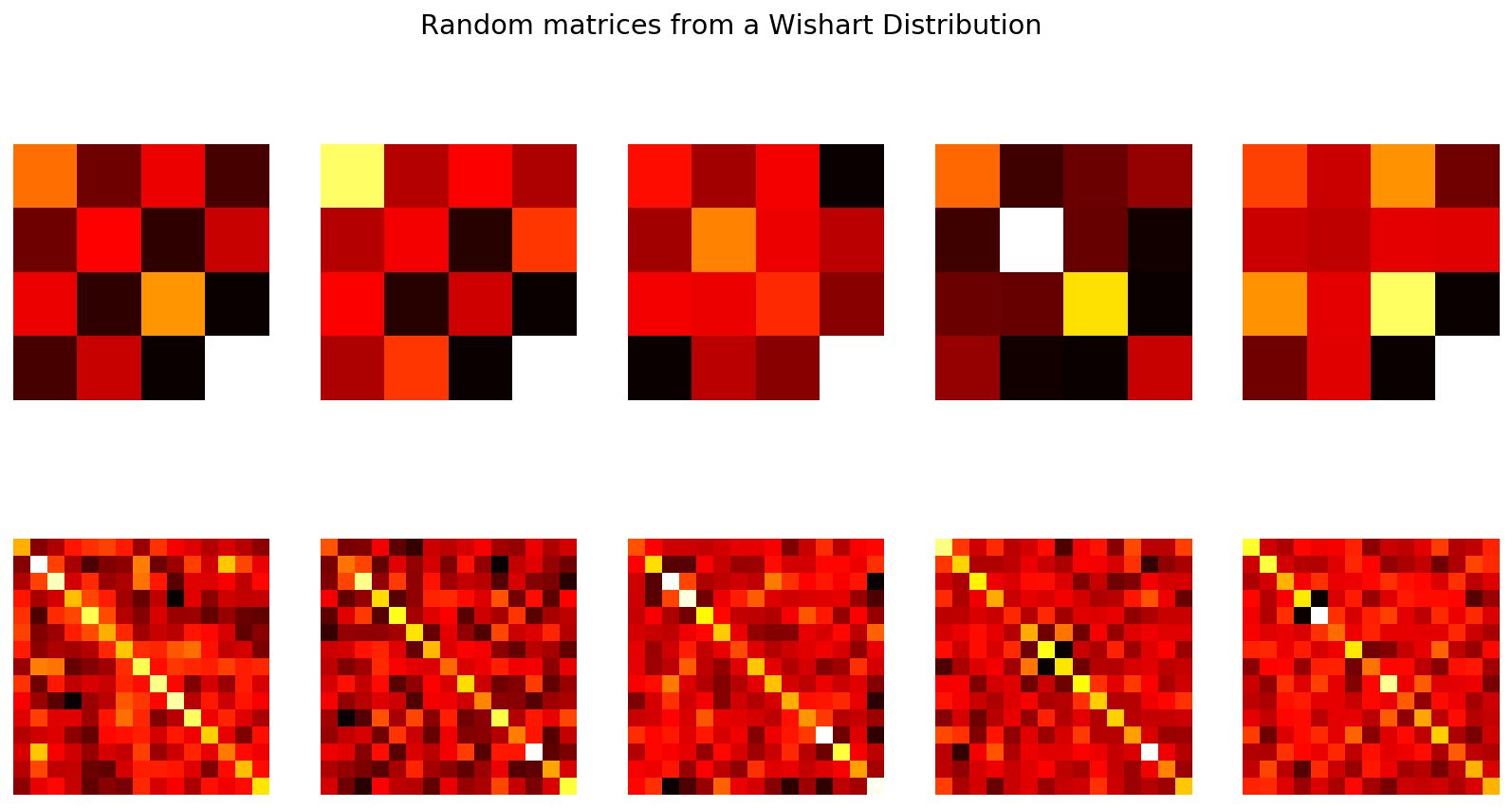One thing to notice is the symmetry of these matrices. The Wishart distribution can be a little troubling to deal with, but we will use it in an example later.

### The Beta distribution¶

You may have seen the term beta in previous code in this book. Often, I was implementing a Beta distribution. The Beta distribution is very useful in Bayesian statistics. A random variable $X$ has a $\text{Beta}$ distribution, with parameters $(\alpha, \beta)$, if its density function is:

$$f_X(x | \; \alpha, \beta ) = \frac{ x^{(\alpha - 1)}(1-x)^{ (\beta - 1) } }{B(\alpha, \beta) }$$

where $B$ is the Beta function (hence the name). The random variable $X$ is only allowed in [0,1], making the Beta distribution a popular distribution for decimal values, probabilities and proportions. The values of $\alpha$ and $\beta$, both positive values, provide great flexibility in the shape of the distribution. Below we plot some distributions:

In :
reset_sess()

params = [(2, 5), (1, 1), (0.5, 0.5), (5, 5), (20, 4), (5, 1)]
x = tf.cast(tf.linspace(start=0.01 ,stop=.99, num=100), dtype=tf.float32)

plt.figure(figsize(12.5, 7))
for alpha, beta in params:
[
y_,
x_
] = evaluate([
tfd.Beta(float(alpha), float(beta)).prob(x),
x,
])
lines = plt.plot(x_, y_, label = "(%.1f,%.1f)"%(alpha, beta), lw = 3)
plt.fill_between(x_, 0, y_, alpha = 0.2, color = lines.get_color())
plt.autoscale(tight=True)
plt.ylim(0)
plt.legend(title=r"$\alpha, \beta$ - parameters");

Device mapping:
/job:localhost/replica:0/task:0/device:XLA_CPU:0 -> device: XLA_CPU device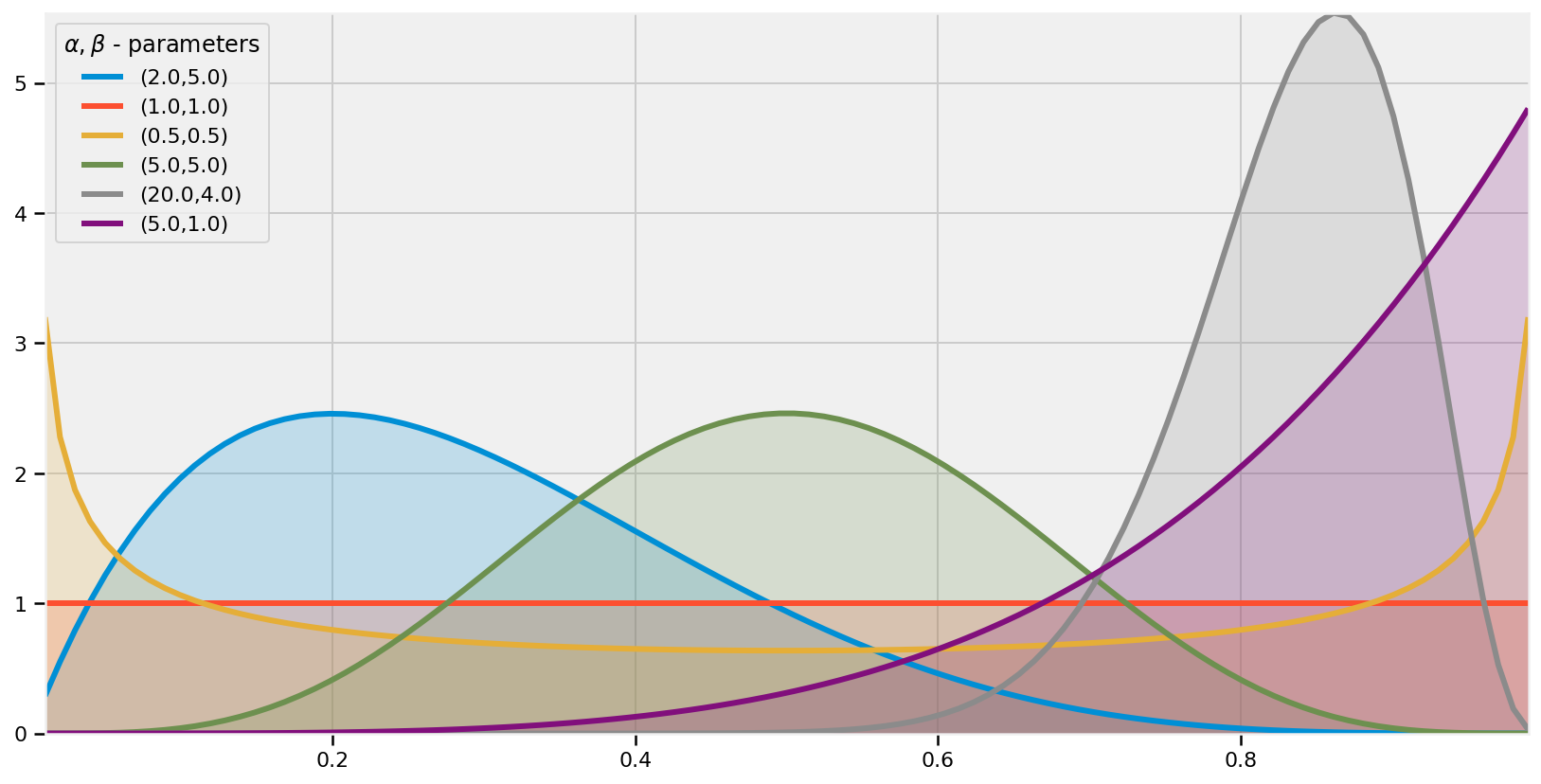One thing I'd like the reader to notice is the presence of the flat distribution above, specified by parameters $(1,1)$. This is the Uniform distribution. Hence the Beta distribution is a generalization of the Uniform distribution, something we will revisit many times.

There is an interesting connection between the Beta distribution and the Binomial distribution. Suppose we are interested in some unknown proportion or probability $p$. We assign a $\text{Beta}(\alpha, \beta)$ prior to $p$. We observe some data generated by a Binomial process, say $X \sim \text{Binomial}(N, p)$, with $p$ still unknown. Then our posterior is again a Beta distribution, i.e. $p | X \sim \text{Beta}( \alpha + X, \beta + N -X )$. Succinctly, one can relate the two by "a Beta prior with Binomial observations creates a Beta posterior". This is a very useful property, both computationally and heuristically.

In light of the above two paragraphs, if we start with a $\text{Beta}(1,1)$ prior on $p$ (which is a Uniform), observe data $X \sim \text{Binomial}(N, p)$, then our posterior is $\text{Beta}(1 + X, 1 + N - X)$.

## Example: Bayesian Multi-Armed Bandits¶

Adapted from an example by Ted Dunning of MapR Technologies

Suppose you are faced with $N$ slot machines (colourfully called multi-armed bandits). Each bandit has an unknown probability of distributing a prize (assume for now the prizes are the same for each bandit, only the probabilities differ). Some bandits are very generous, others not so much. Of course, you don't know what these probabilities are. By only choosing one bandit per round, our task is devise a strategy to maximize our winnings.

Of course, if we knew the bandit with the largest probability, then always picking this bandit would yield the maximum winnings. So our task can be phrased as "Find the best bandit, and as quickly as possible".

The task is complicated by the stochastic nature of the bandits. A suboptimal bandit can return many winnings, purely by chance, which would make us believe that it is a very profitable bandit. Similarly, the best bandit can return many duds. Should we keep trying losers then, or give up?

A more troublesome problem is, if we have found a bandit that returns pretty good results, do we keep drawing from it to maintain our pretty good score, or do we try other bandits in hopes of finding an even-better bandit? This is the exploration vs. exploitation dilemma.

### Applications¶

The Multi-Armed Bandit problem at first seems very artificial, something only a mathematician would love, but that is only before we address some applications:

• Internet display advertising: companies have a suite of potential ads they can display to visitors, but the company is not sure which ad strategy to follow to maximize sales. This is similar to A/B testing, but has the added advantage of naturally minimizing strategies that do not work (and generalizes to A/B/C/D... strategies)
• Ecology: animals have a finite amount of energy to expend, and following certain behaviours has uncertain rewards. How does the animal maximize its fitness?
• Finance: which stock option gives the highest return, under time-varying return profiles.
• Clinical trials: a researcher would like to find the best treatment, out of many possible treatment, while minimizing losses.
• Psychology: how does punishment and reward affect our behaviour? How do humans learn?

Many of these questions above are fundamental to the application's field.

It turns out the optimal solution is incredibly difficult, and it took decades for an overall solution to develop. There are also many approximately-optimal solutions which are quite good. The one I wish to discuss is one of the few solutions that can scale incredibly well. The solution is known as Bayesian Bandits.

### A Proposed Solution¶

Any proposed strategy is called an online algorithm (not in the internet sense, but in the continuously-being-updated sense), and more specifically a reinforcement learning algorithm. The algorithm starts in an ignorant state, where it knows nothing, and begins to acquire data by testing the system. As it acquires data and results, it learns what the best and worst behaviours are (in this case, it learns which bandit is the best). With this in mind, perhaps we can add an additional application of the Multi-Armed Bandit problem:

• Psychology: how does punishment and reward affect our behaviour? How do humans learn?

The Bayesian solution begins by assuming priors on the probability of winning for each bandit. In our vignette we assumed complete ignorance of these probabilities. So a very natural prior is the flat prior over 0 to 1. The algorithm proceeds as follows:

For each round:

1. Sample a random variable $X_b$ from the prior of bandit $b$, for all $b$.
2. Select the bandit with largest sample, i.e. select $B = \text{argmax}\;\; X_b$.
3. Observe the result of pulling bandit $B$, and update your prior on bandit $B$.
4. Return to 1.

That's it. Computationally, the algorithm involves sampling from $N$ distributions. Since the initial priors are $\text{Beta}(\alpha=1,\beta=1)$ (a uniform distribution), and the observed result $X$ (a win or loss, encoded 1 and 0 respectfully) is Binomial, the posterior is a $\text{Beta}(\alpha=1+X,\beta=1+1-X)$.

To answer our question from before, this algorithm suggests that we should not discard losers, but we should pick them at a decreasing rate as we gather confidence that there exist better bandits. This follows because there is always a non-zero chance that a loser will achieve the status of $B$, but the probability of this event decreases as we play more rounds (see figure below).

Below we implement Bayesian Bandits using two classes, Bandits that defines the slot machines, and BayesianStrategy which implements the above learning strategy.

In :
reset_sess()

class Bandits(object):
"""
This class represents N bandits machines.

parameters:
arm_true_payout_probs: a (n,) Numpy array of probabilities >0, <1.

methods:
pull( i ): return the results, 0 or 1, of pulling
the ith bandit.
"""
def __init__(self, arm_true_payout_probs):
self._arm_true_payout_probs = tf.convert_to_tensor(
arm_true_payout_probs,
preferred_dtype=tf.float32,
name='arm_true_payout_probs')
self._uniform = tfd.Uniform(low=0., high=1.)
assert self._arm_true_payout_probs.shape.is_fully_defined()
self._shape = np.array(
self._arm_true_payout_probs.shape.as_list(),
dtype=np.int32)
self._dtype = tf.convert_to_tensor(
arm_true_payout_probs,
preferred_dtype=tf.float32).dtype.base_dtype

@property
def dtype(self):
return self._dtype

@property
def shape(self):
return self._shape

def pull(self, arm):
return (self._uniform.sample(self.shape[:-1]) <
self._arm_true_payout_probs[..., arm])

def optimal_arm(self):
return tf.argmax(
self._arm_true_payout_probs,
axis=-1,
name='optimal_arm')

Device mapping:
/job:localhost/replica:0/task:0/device:XLA_CPU:0 -> device: XLA_CPU device


In :
class BayesianStrategy(object):
"""
Implements a online, learning strategy to solve
the Multi-Armed Bandit problem.

parameters:
bandits: a Bandit class with .pull method

methods:
sample_bandits(n): sample and train on n pulls.
"""

def __init__(self, bandits):
self.bandits = bandits
dtype = bandits._dtype
self.wins_var = tf.Variable(
initial_value=tf.zeros(self.bandits.shape, dtype))
self.trials_var = tf.Variable(
initial_value=tf.zeros(self.bandits.shape, dtype))

def sample_bandits(self, n=1):
return tf.while_loop(
cond=lambda *args: True,
body=self._one_trial,
loop_vars=(tf.identity(self.wins_var),
tf.identity(self.trials_var)),
maximum_iterations=n,
parallel_iterations=1)

def make_posterior(self, wins, trials):
return tfd.Beta(concentration1=1. + wins,
concentration0=1. + trials - wins)

def _one_trial(self, wins, trials):
# sample from the bandits's priors, and select the largest sample
rv_posterior_payout = self.make_posterior(wins, trials)
posterior_payout = rv_posterior_payout.sample()
choice = tf.argmax(posterior_payout, axis=-1)

# Update trials.
one_hot_choice = tf.reshape(
tf.one_hot(
indices=tf.reshape(choice, shape=[-1]),
depth=self.bandits.shape[-1],
dtype=self.trials_var.dtype.base_dtype),
shape=tf.shape(wins))
trials = tf.assign_add(self.trials_var, one_hot_choice)

# Update wins.
result = self.bandits.pull(choice)
update = tf.where(result, one_hot_choice, tf.zeros_like(one_hot_choice))
wins = tf.assign_add(self.wins_var, update)

return wins, trials


Below we visualize the learning of the Bayesian Bandit solution.

In :
reset_sess()

hidden_prob_ = np.array([0.85, 0.60, 0.75])
bandits = Bandits(hidden_prob_)
bayesian_strat = BayesianStrategy(bandits)

draw_samples_ = np.array([1, 1, 3, 10, 10, 25, 50, 100, 200, 600])

def plot_priors(bayesian_strategy, prob, wins, trials,
lw = 3, alpha = 0.2, plt_vlines = True):
## plotting function
for i in range(prob.shape):
posterior_dists = tf.cast(tf.linspace(start=0.001 ,stop=.999, num=200), dtype=tf.float32)
y = tfd.Beta(concentration1 = tf.cast((1+wins[i]), dtype=tf.float32) ,
concentration0 = tf.cast((1 + trials[i] - wins[i]), dtype=tf.float32))
y_prob_i = y.prob(tf.cast(prob[i], dtype=tf.float32))
y_probs = y.prob(tf.cast(posterior_dists, dtype=tf.float32))
[
posterior_dists_,
y_probs_,
y_prob_i_,
] = evaluate([
posterior_dists,
y_probs,
y_prob_i,
])

p = plt.plot(posterior_dists_, y_probs_, lw = lw)
c = p.get_markeredgecolor()
plt.fill_between(posterior_dists_, y_probs_,0, color = c, alpha = alpha,
label="underlying probability: %.2f" % prob[i])
if plt_vlines:
plt.vlines(prob[i], 0, y_prob_i_ ,
colors = c, linestyles = "--", lw = 2)
plt.autoscale(tight = "True")
plt.title("Posteriors After %d pull" % N_pulls +\
"s"*(N_pulls > 1))
plt.autoscale(tight=True)
return

plt.figure(figsize(11.0, 12))
for j,i in enumerate(draw_samples_):
plt.subplot(5, 2, j+1)
evaluate(tf.global_variables_initializer())
[wins_, trials_] = evaluate(bayesian_strat.sample_bandits(i))
N_pulls = int(draw_samples_.cumsum()[j])
plot_priors(bayesian_strat, hidden_prob_, wins=wins_, trials=trials_)
#plt.legend()
plt.autoscale(tight = True)
plt.tight_layout()

Device mapping:
/job:localhost/replica:0/task:0/device:XLA_CPU:0 -> device: XLA_CPU device

WARNING:tensorflow:From <ipython-input-3-e53af945c0d7>:51: where (from tensorflow.python.ops.array_ops) is deprecated and will be removed in a future version.
Instructions for updating:
Use tf.where in 2.0, which has the same broadcast rule as np.where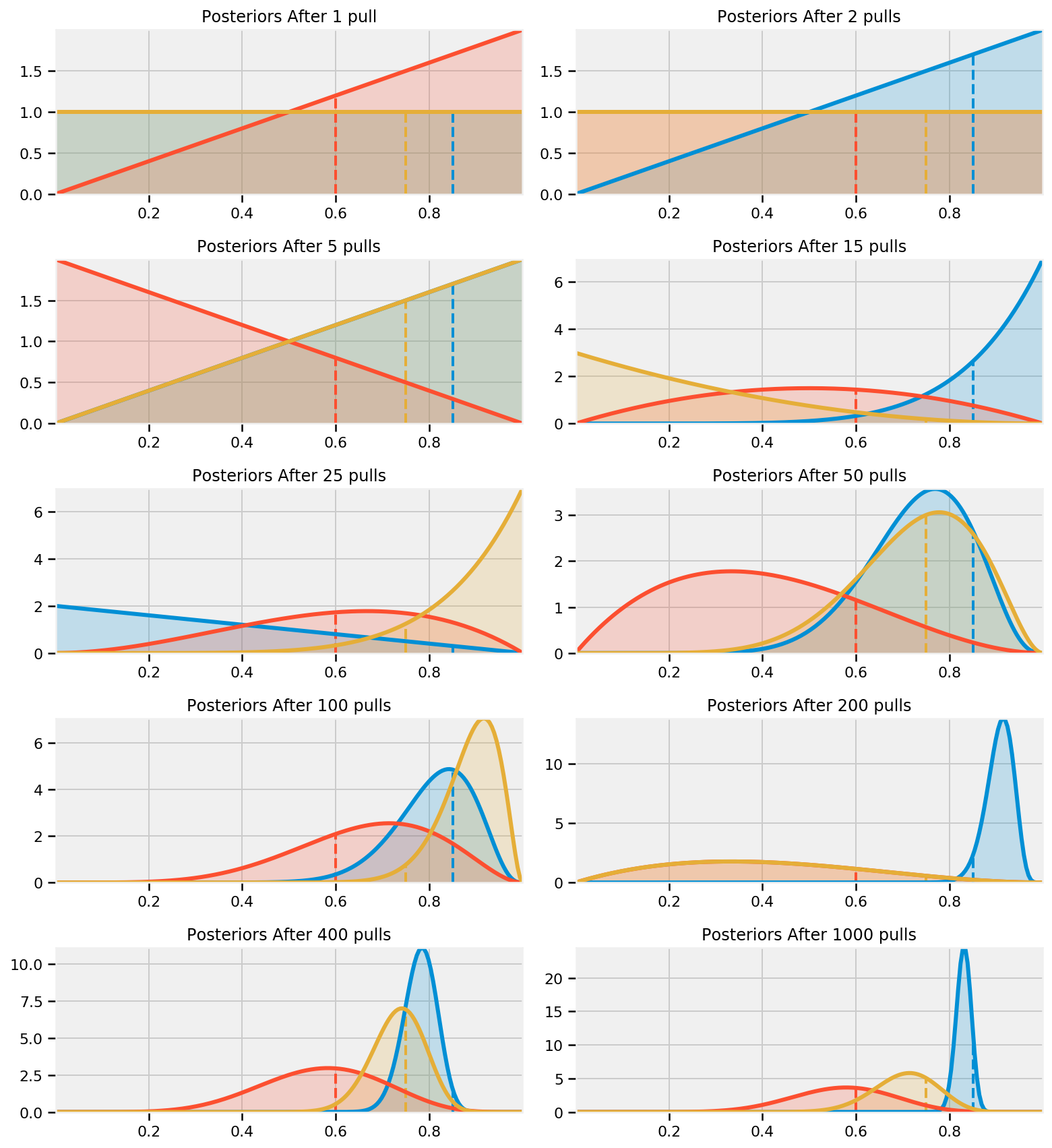Note that we don't really care how accurate we become about the inference of the hidden probabilities — for this problem we are more interested in choosing the best bandit (or more accurately, becoming more confident in choosing the best bandit). For this reason, the distribution of the red bandit is very wide (representing ignorance about what that hidden probability might be) but we are reasonably confident that it is not the best, so the algorithm chooses to ignore it.

From the above, we can see that after 1000 pulls, the majority of the "blue" function leads the pack, hence we will almost always choose this arm. This is good, as this arm is indeed the best.

Below is a D3 app that demonstrates our algorithm updating/learning three bandits. The first figure are the raw counts of pulls and wins, and the second figure is a dynamically updating plot. I encourage you to try to guess which bandit is optimal, prior to revealing the true probabilities, by selecting the arm buttons.

In :
# Getting the HTML file for the simulated Bayesian Bandits
reset_sess()

import wget
url = 'https://raw.githubusercontent.com/CamDavidsonPilon/Probabilistic-Programming-and-Bayesian-Methods-for-Hackers/master/Chapter6_Priorities/BanditsD3.html'
filename = wget.download(url)
filename

Device mapping:
/job:localhost/replica:0/task:0/device:XLA_CPU:0 -> device: XLA_CPU device


Out:
'BanditsD3 (3).html'
In :
from IPython.core.display import HTML

#try executing the below command twice if the first time doesn't work
HTML(filename = "BanditsD3.html")

Out:

Rewards

0

Pulls

0

Reward/Pull Ratio

0

Deviations of the observed ratio from the highest probability is a measure of performance. For example, in the long run, we can attain the reward/pull ratio of the maximum bandit probability if we are optimal. Long-term realized ratios less than the maximum represent inefficiencies. (Realized ratios larger than the maximum probability is due to randomness, and will eventually fall below).

### A Measure of Good¶

We need a metric to calculate how well we are doing. Recall the absolute best we can do is to always pick the bandit with the largest probability of winning. Denote this best bandit's probability by $w_{opt}$. Our score should be relative to how well we would have done had we chosen the best bandit from the beginning. This motivates the total regret of a strategy, defined: \begin{align} R_T & = \sum_{i=1}^{T} \left( w_{opt} - w_{B(i)} \right)\\ & = Tw^* - \sum_{i=1}^{T} \; w_{B(i)} \end{align}

where $w_{B(i)}$ is the probability of a prize of the chosen bandit in the $i$ round. A total regret of 0 means the strategy is matching the best possible score. This is likely not possible, as initially our algorithm will often make the wrong choice. Ideally, a strategy's total regret should flatten as it learns the best bandit. (Mathematically, we achieve $w_{B(i)}=w_{opt}$ often)

Below we plot the total regret of this simulation, including the scores of some other strategies:

1. Random: randomly choose a bandit to pull. If you can't beat this, just stop.
2. Largest Bayesian credible bound: pick the bandit with the largest upper bound in its 95% credible region of the underlying probability.
3. Bayes-UCB algorithm: pick the bandit with the largest score, where score is a dynamic quantile of the posterior (see  )
4. Mean of posterior: choose the bandit with the largest posterior mean. This is what a human player (sans computer) would likely do.
5. Largest proportion: pick the bandit with the current largest observed proportion of winning.

The code for these are in the other_strats.py, where you can implement your own very easily.

In :
url = 'https://raw.githubusercontent.com/CamDavidsonPilon/Probabilistic-Programming-and-Bayesian-Methods-for-Hackers/master/Chapter6_Priorities/other_strats.py'
filename = wget.download(url)
filename

Out:
'other_strats.py'
In :
plt.figure(figsize(12.5, 5))
from other_strats import *

#define a harder problem
hidden_prob = np.array([0.15, 0.2, 0.1, 0.05])
bandits = Bandits(hidden_prob)

#define regret
def regret(probabilities, choices):
w_opt = probabilities.max()
return (w_opt - probabilities[choices.astype(int)]).cumsum()

#create new strategies
strategies= [upper_credible_choice,
bayesian_bandit_choice,
ucb_bayes ,
max_mean,
random_choice]
algos = []
for strat in strategies:
algos.append(GeneralBanditStrat(bandits, strat))

#train 10000 times
for strat in algos:
strat.sample_bandits(10000)

#test and plot
for i,strat in enumerate(algos):
_regret = regret(hidden_prob, strat.choices)
plt.plot(_regret, label = strategies[i].__name__, lw = 3)

plt.title(r"Total Regret of Bayesian Bandits Strategy vs. Random guessing")
plt.xlabel(r"Number of pulls")
plt.ylabel(r"Regret after $n$ pulls");
plt.legend(loc = "upper left");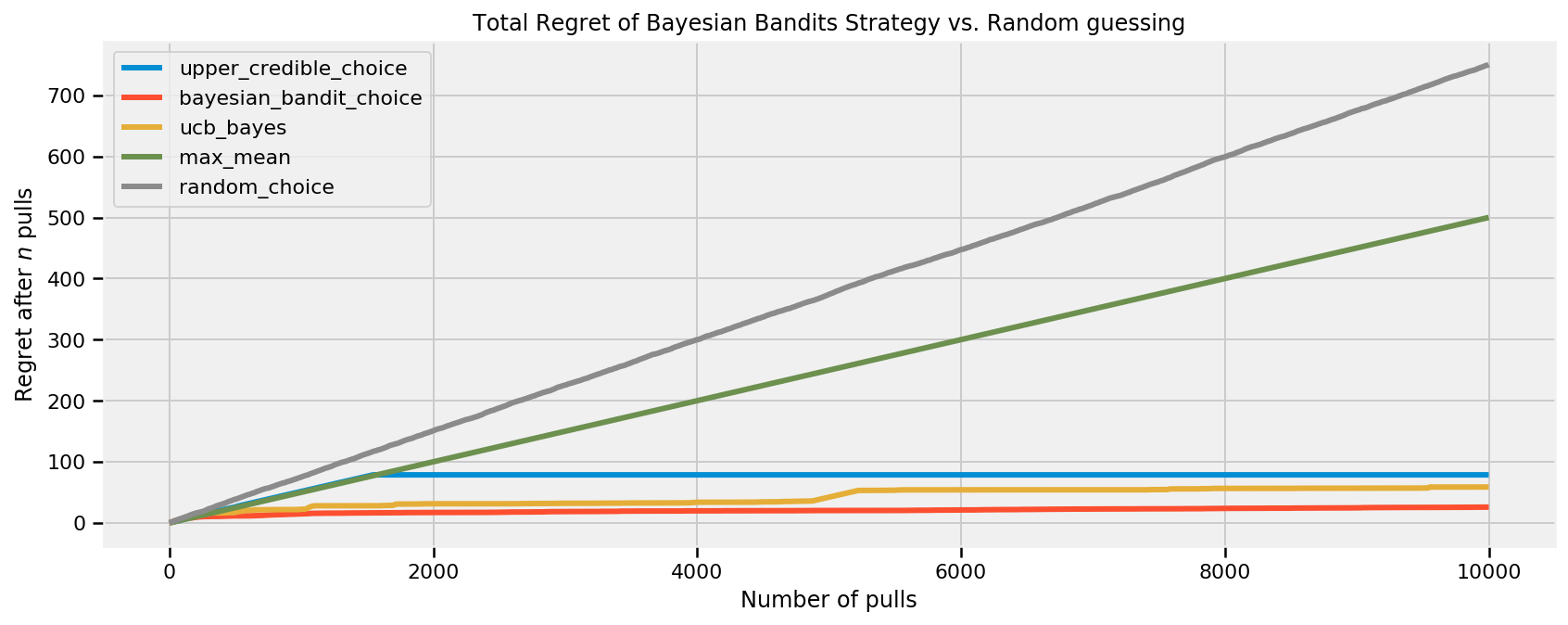Like we wanted, Bayesian bandits and other strategies have decreasing rates of regret, representing we are achieving optimal choices. To be more scientific so as to remove any possible luck in the above simulation, we should instead look at the expected total regret:

$$\bar{R}_T = E[ R_T ]$$

It can be shown that any sub-optimal strategy's expected total regret is bounded below logarithmically. Formally,

$$E[R_T] = \Omega \left( \;\log(T)\; \right)$$

Thus, any strategy that matches logarithmic-growing regret is said to "solve" the Multi-Armed Bandit problem .

Using the Law of Large Numbers, we can approximate Bayesian Bandit's expected total regret by performing the same experiment many times (500 times, to be fair):

In :
# This can be slow, so I recommend NOT running it.
# Estimated time for Graph Mode: 16 minutes.

trials = tf.constant(500)
expected_total_regret = tf.zeros((10000, 3))

[
trials_,
expected_total_regret_,
] = evaluate([
trials,
expected_total_regret,
])

for i_strat, strat in enumerate(strategies[:-2]):
for i in range(trials_):
general_strat = GeneralBanditStrat(bandits, strat)
general_strat.sample_bandits(10000)
_regret = regret(hidden_prob, general_strat.choices)
expected_total_regret_[:,i_strat] += _regret
plt.plot(expected_total_regret_[:,i_strat]/trials_, lw =3, label = strat.__name__)

plt.title("Expected Total Regret of Multi-armed Bandit strategies")
plt.xlabel("Number of pulls")
plt.ylabel("Exepected Total Regret \n after $n$ pulls");
plt.legend(loc = "upper left");
`